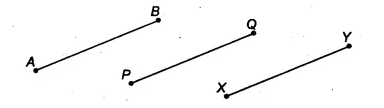# Which of the following statements are true and which are false? Give reasons for your answers

Which of the following statements are true and which are false? Give reasons for your answers
(i) Only one line can pass through a single point.
(ii) There are an infinite number of lines which pass through two distinct points.
(iii) A terminated line can be produced indefinitely on both sides.
(iv) If two circles are equal, then their radii are equal.
(v) In figure, if AB – PQ and PQ = XY, then AB = XY.